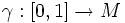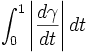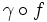# Arc-length functional

Fix a Riemannian manifold$M$. The arc-length functional is a map from the space of piecewise smooth curves in the manifold, to real numbers, defined as follows. The arc-length of a curve$\gamma:[0,1] \to M$ is:$\int_0^1 \left|\frac{d\gamma}{dt}\right| dt$
Interestingly, the arc-length is independent of the parametrization of the curve. In other words , if$f:[0,1] \to [0,1]$ is an increasing function, then$\gamma \circ f$ has the same arc-length as$\gamma$.